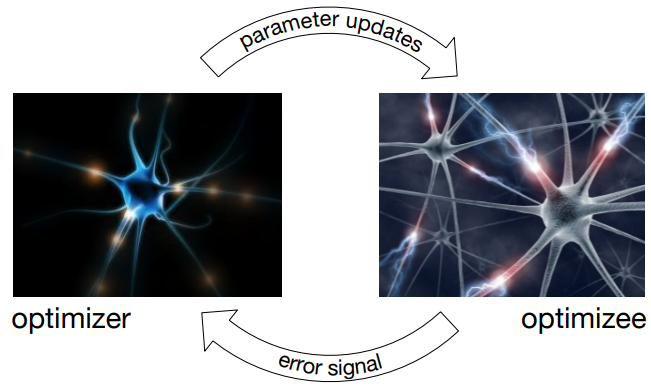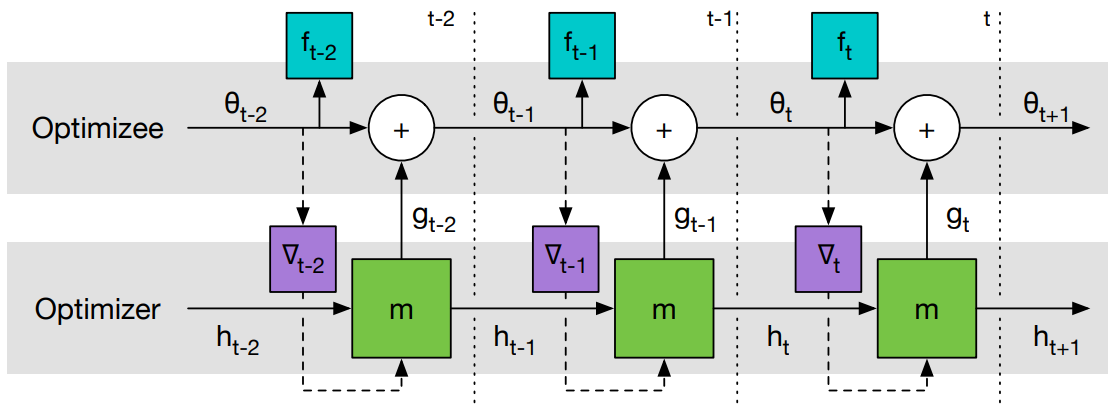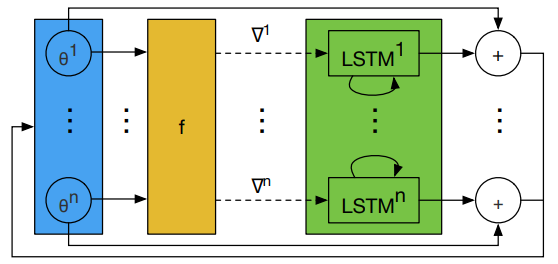# Learning to Learn¶

Learning to Learn by Gradient Descent by Gradient Descent 提出了一种全新的优化策略， 用 LSTM 替代传统优化方法学习一个针对特定任务的优化器。

## 1 问题提出¶

$\theta^{*}=\operatorname{argmin}_{\theta \in \Theta} f(\theta)$

$\theta_{t+1}=\theta_{t}-\alpha \nabla f\left(\theta_{t}\right)$

$\theta_{t+1}=\theta_{t}+g_{t}\left(\nabla f\left(\theta_{t}\right), \phi\right)$

optimizer $$g$$ 的更新则由 $$f$$, $$\nabla f$$$$\phi$$ 决定。

## 2 Learning to Learn¶

### 2.1 学习机制¶$\mathcal{L}(\phi)=\mathbb{E}_{f}\left[\sum_{t=1}^{T} \omega_{t} f\left(\theta_{t}\right)\right]$

\begin{split} \begin{aligned} & \theta_{t+1}=\theta_{t}+g_{t} \\ & {\left[g_{t}, h_{t+1}\right]=\operatorname{LSTM}\left(\nabla_{t}, h_{t}, \phi\right)} \end{aligned} \end{split}

$$\omega_{t} \in \mathbb{R}_{\geq 0}$$ 是各个优化时刻的任意权重， $$\nabla_{t}=\nabla_{\theta} f\left(\theta_{t}\right)$$中 optimizer 选用了 LSTM 。 从 LSTM 优化器的设计来看， 几乎没有加入任何先验的人为经验。 优化器本身的参数 $$\phi$$ 即 LSTM 的参数， 这个优化器的参数代表了更新策略。

### 2.2 Coordinatewise LSTM optimizer¶

LSTM 需要优化的参数相对较多。 因此， 设计了一个优化器 $$m$$，它可以对目标函数的每个参数分量进行操作。 具体而言，每次只对 optimizee 的一个参数分量 $$\theta_{i}$$ 进行优化， 这样只需要维持一个很小的 optimizer 就可以完成工作。### 2.3 预处理和后处理¶

$\begin{split} \nabla^{k} \rightarrow \begin{cases}\left(\frac{\log (|\nabla|)}{p}, \operatorname{sgn}(\nabla)\right) & \text { if }|\nabla| \geq e^{-p} \\ \left(-1, e^{p} \nabla\right) & \text { otherwise }\end{cases} \end{split}$